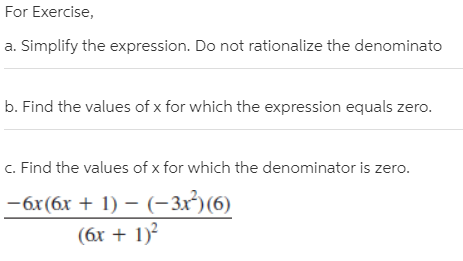# For Exercise, a. Simplify the expression. Do not rationalize the denominato b. Find the values of x for which the expression equals zero. c. Find the values of x for which the denominator is zero. -6x(6x + 1) – (-3x²)(6) (6x + 1)?

Questionhelp_outlineImage TranscriptioncloseFor Exercise, a. Simplify the expression. Do not rationalize the denominato b. Find the values of x for which the expression equals zero. c. Find the values of x for which the denominator is zero. -6x(6x + 1) – (-3x²)(6) (6x + 1)? fullscreen